# RD Sharma Solutions Class 12 Probability Exercise 31.7

RD Sharma Solutions for Class 12 Maths Chapter 31 Probability Exercise 31.7 are given here for students who aspire to kick start preparations for their board examinations. These solutions contain shortcut tips and tricks to help students achieve high marks in the exams. Further, students are recommended to practise the RD Sharma Class 12 Solutions regularly to attain a strong grip over various solving methodologies. Also, they can now download these solutions in PDF format for free, from the link provided below.

## Download PDF of RD Sharma Solutions for Class 12 Maths Chapter 31 Exercise 7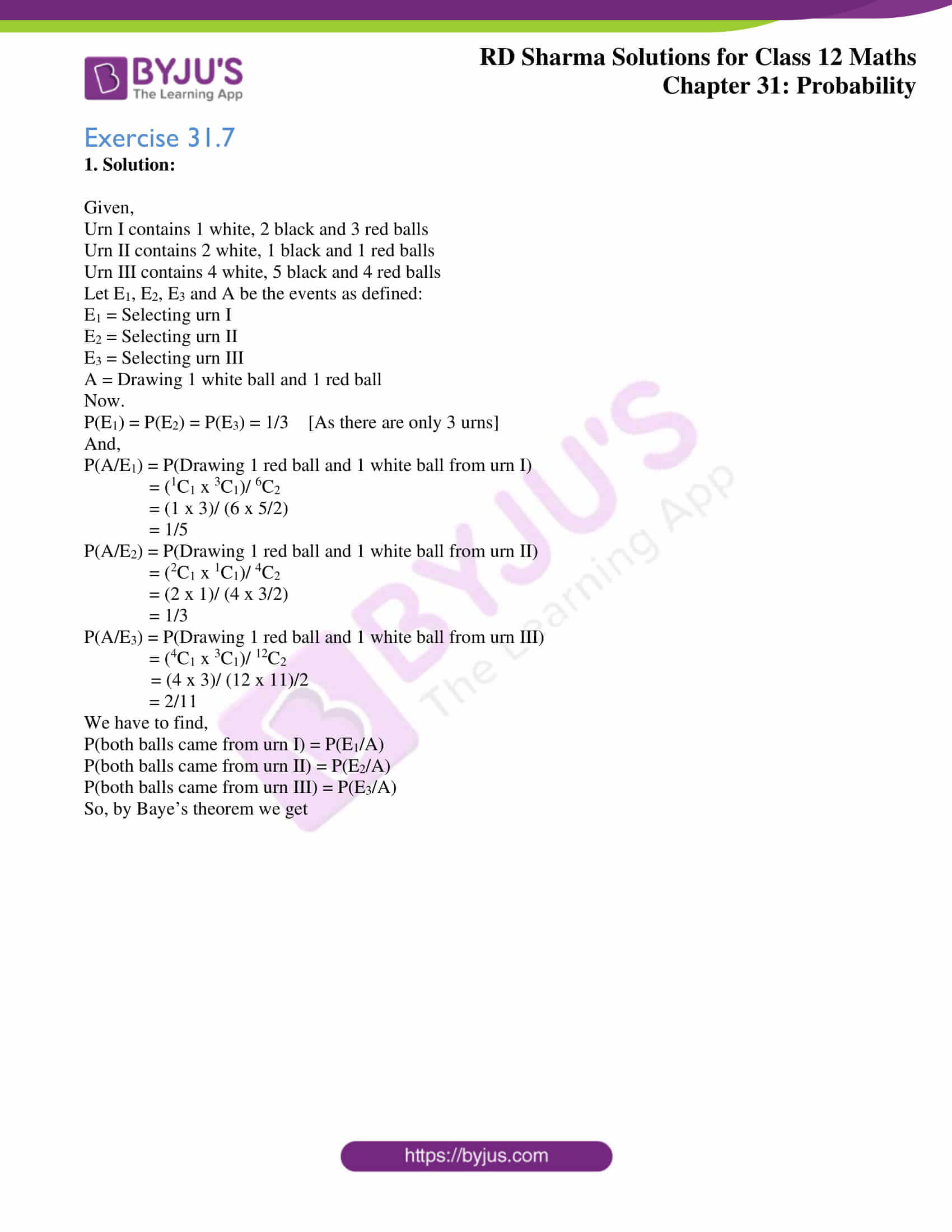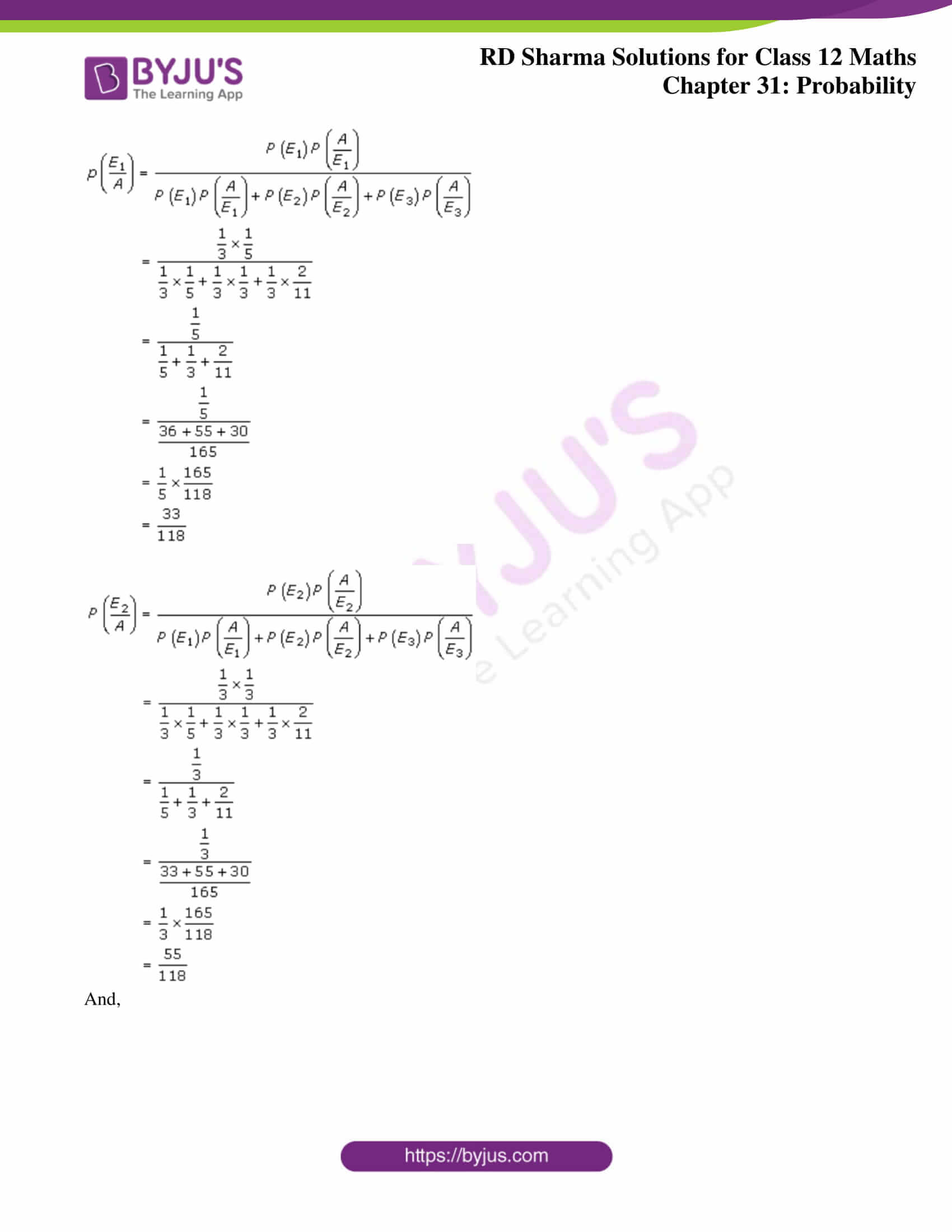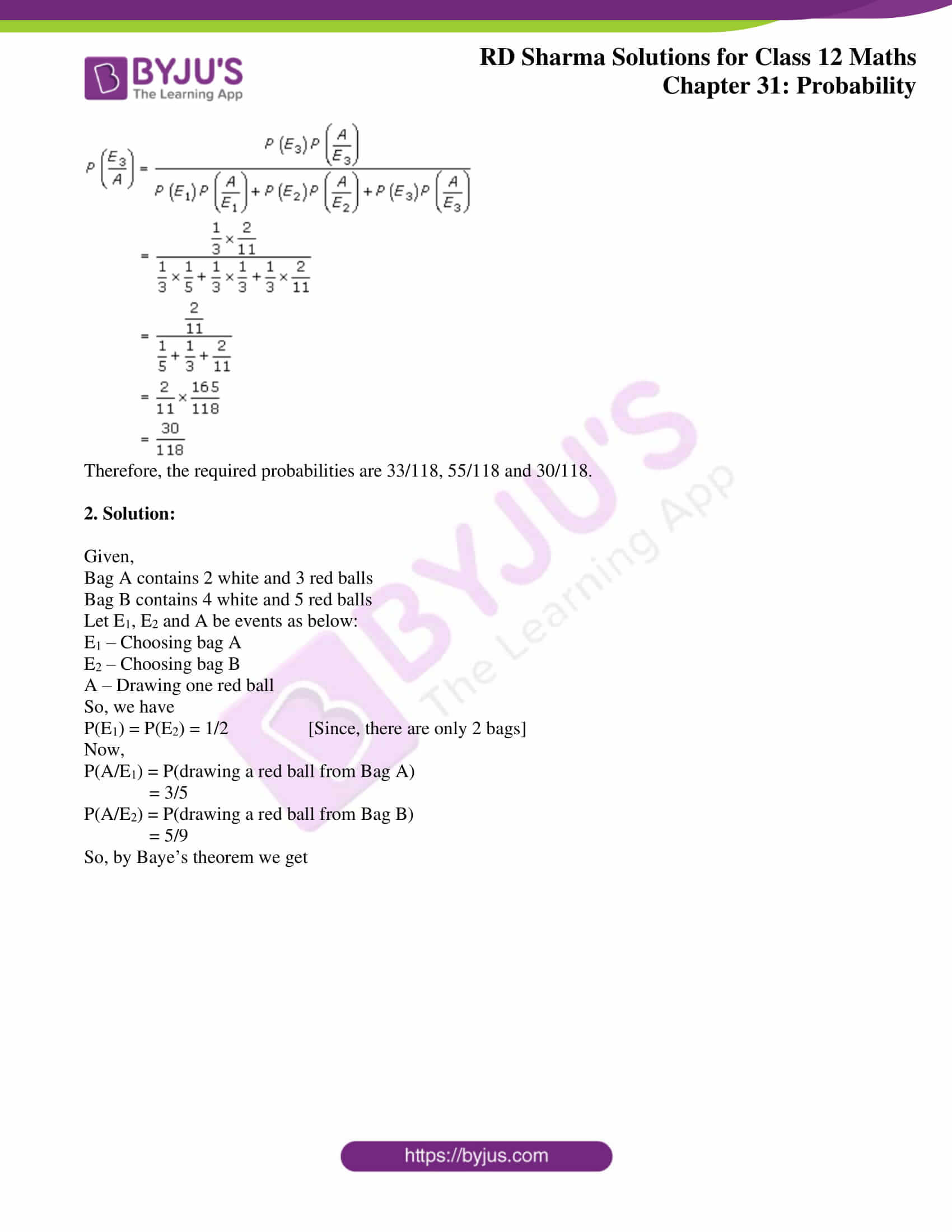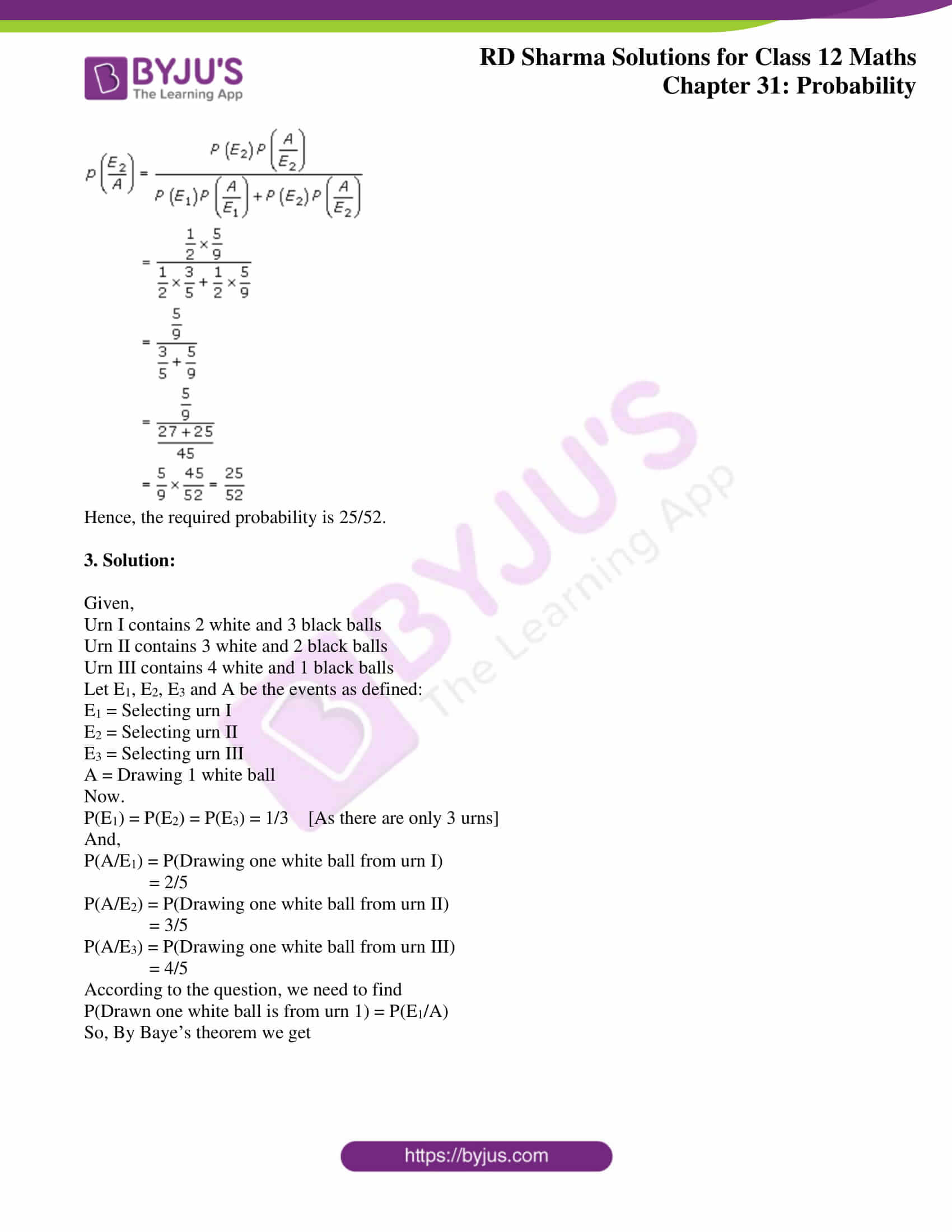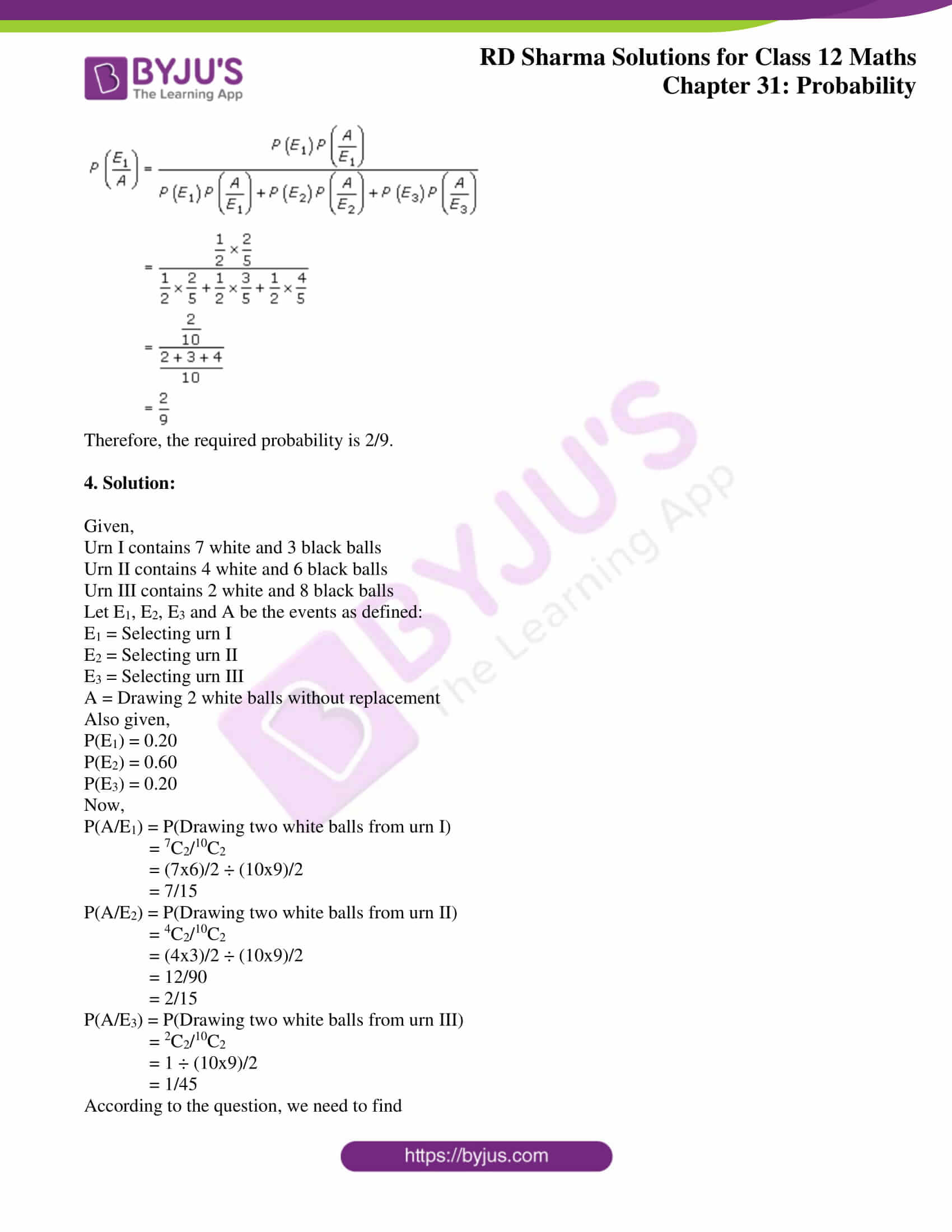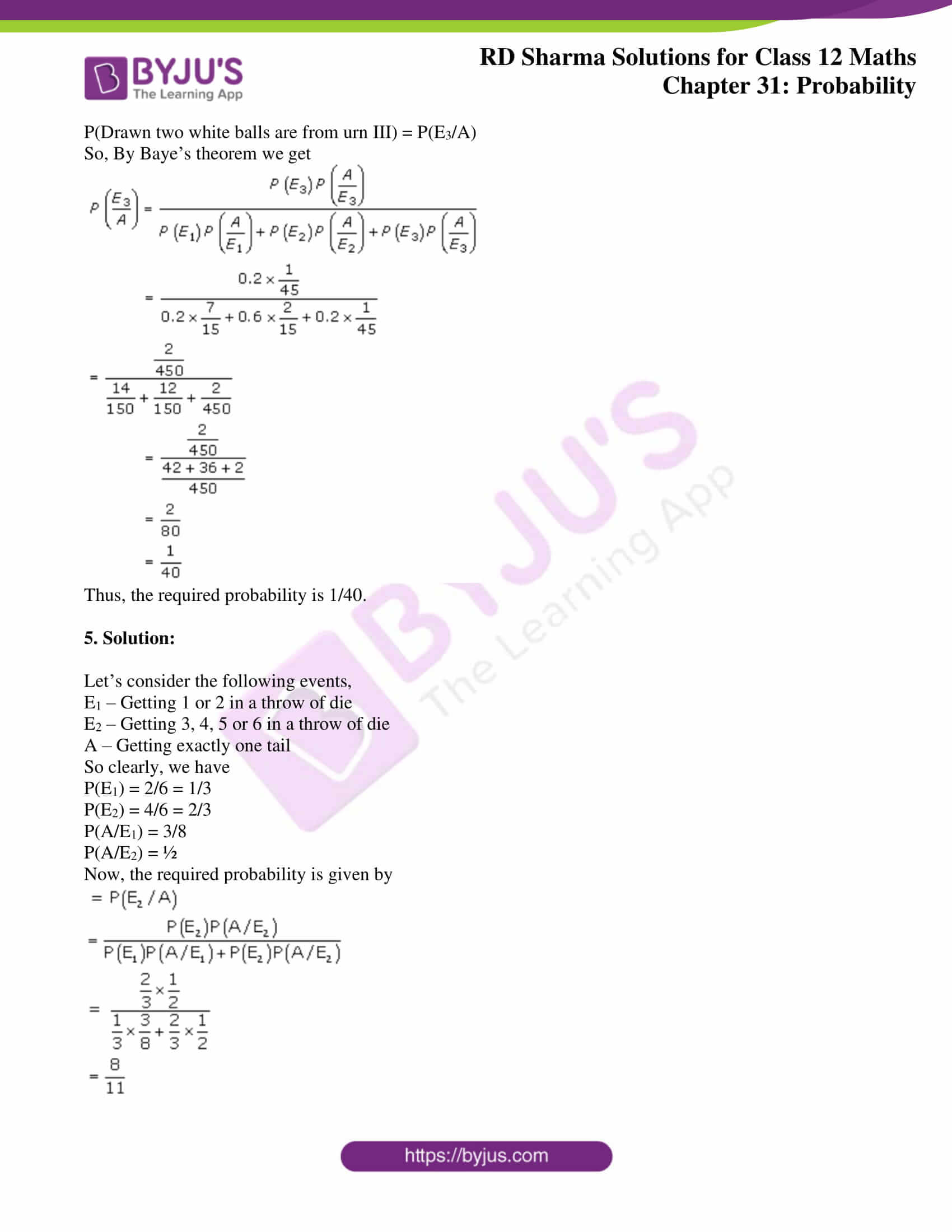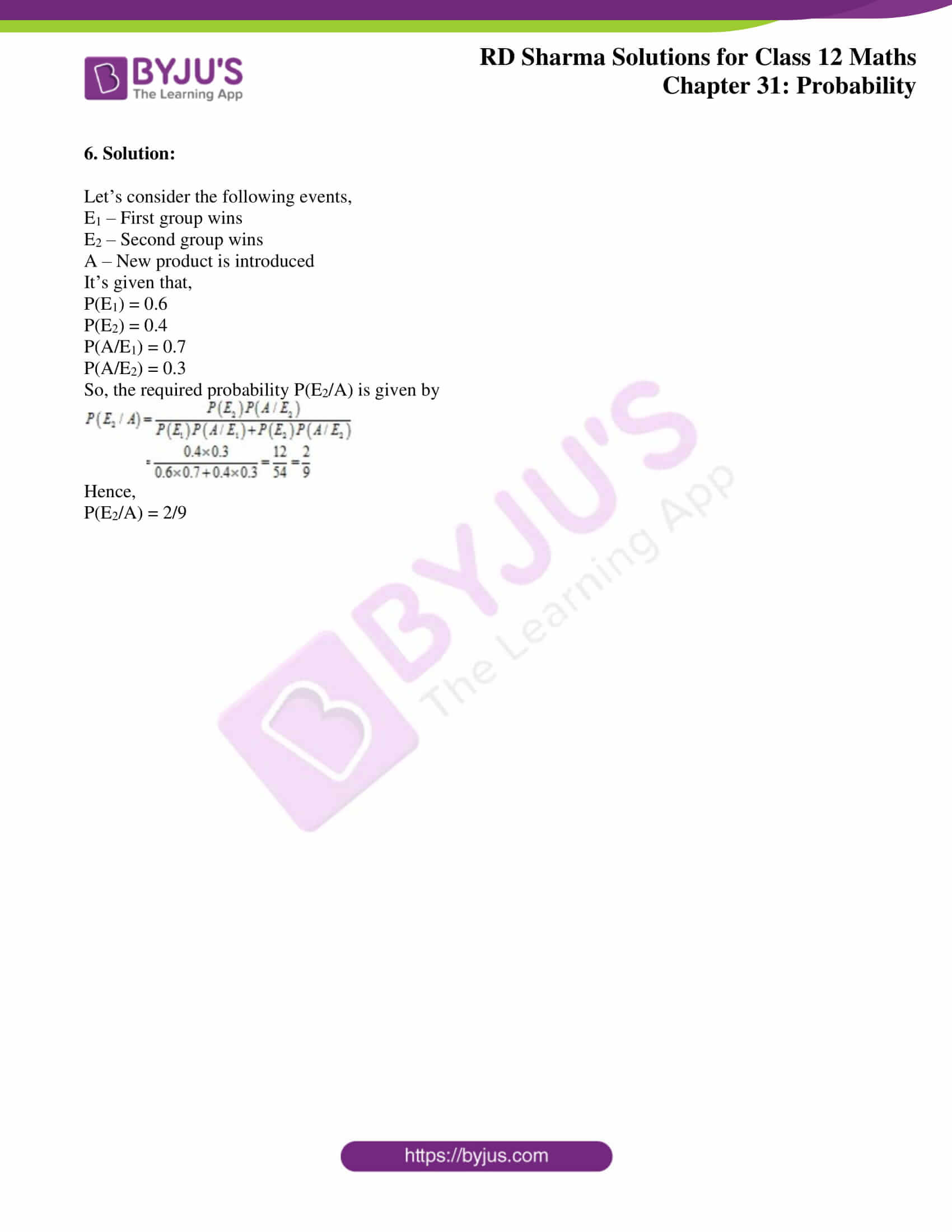### Access RD Sharma Solutions for Class 12 Maths Chapter 31 Exercise 7

Exercise 31.7

1. Solution:

Given,

Urn I contains 1 white, 2 black and 3 red balls

Urn II contains 2 white, 1 black and 1 red balls

Urn III contains 4 white, 5 black and 4 red balls

Let E1, E2, E3 and A be the events as defined:

E1 = Selecting urn I

E2 = Selecting urn II

E3 = Selecting urn III

A = Drawing 1 white ball and 1 red ball

Now.

P(E1) = P(E2) = P(E3) = 1/3 [As there are only 3 urns]

And,

P(A/E1) = P(Drawing 1 red ball and 1 white ball from urn I)

= (1C1 x 3C1)/ 6C2

= (1 x 3)/ (6 x 5/2)

= 1/5

P(A/E2) = P(Drawing 1 red ball and 1 white ball from urn II)

= (2C1 x 1C1)/ 4C2

= (2 x 1)/ (4 x 3/2)

= 1/3

P(A/E3) = P(Drawing 1 red ball and 1 white ball from urn III)

= (4C1 x 3C1)/ 12C2

= (4 x 3)/ (12 x 11)/2

= 2/11

We have to find,

P(both balls came from urn I) = P(E1/A)

P(both balls came from urn II) = P(E2/A)

P(both balls came from urn III) = P(E3/A)

So, by Baye’s theorem we get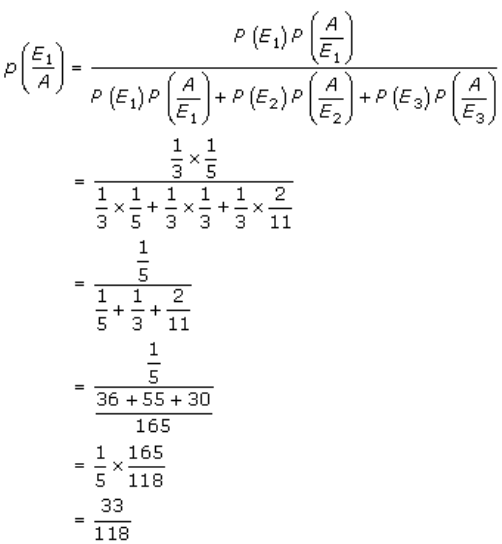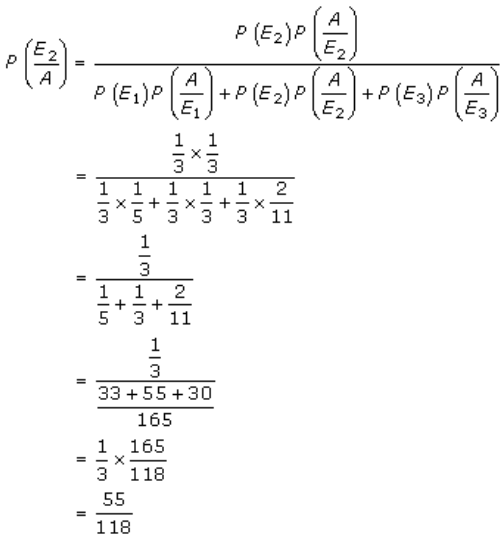And,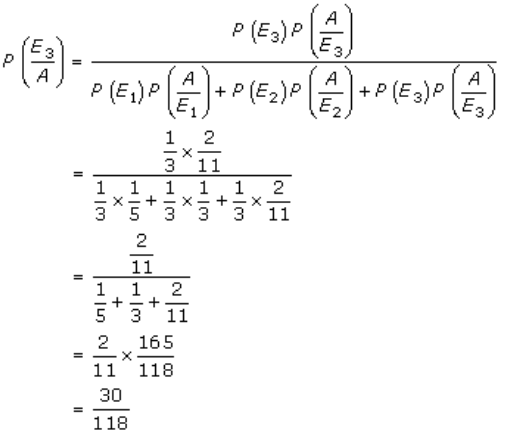Therefore, the required probabilities are 33/118, 55/118 and 30/118.

2. Solution:

Given,

Bag A contains 2 white and 3 red balls

Bag B contains 4 white and 5 red balls

Let E1, E2 and A be events as below:

E1 – Choosing bag A

E2 – Choosing bag B

A – Drawing one red ball

So, we have

P(E1) = P(E2) = 1/2 [Since, there are only 2 bags]

Now,

P(A/E1) = P(drawing a red ball from Bag A)

= 3/5

P(A/E2) = P(drawing a red ball from Bag B)

= 5/9

So, by Baye’s theorem we get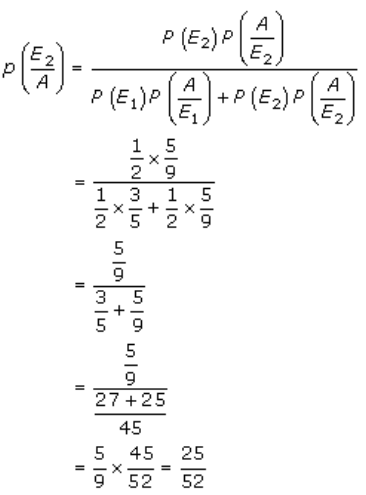Hence, the required probability is 25/52.

3. Solution:

Given,

Urn I contains 2 white and 3 black balls

Urn II contains 3 white and 2 black balls

Urn III contains 4 white and 1 black balls

Let E1, E2, E3 and A be the events as defined:

E1 = Selecting urn I

E2 = Selecting urn II

E3 = Selecting urn III

A = Drawing 1 white ball

Now.

P(E1) = P(E2) = P(E3) = 1/3 [As there are only 3 urns]

And,

P(A/E1) = P(Drawing one white ball from urn I)

= 2/5

P(A/E2) = P(Drawing one white ball from urn II)

= 3/5

P(A/E3) = P(Drawing one white ball from urn III)

= 4/5

According to the question, we need to find

P(Drawn one white ball is from urn 1) = P(E1/A)

So, By Baye’s theorem we get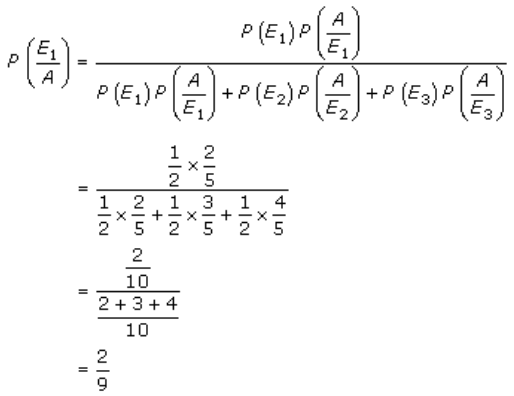Therefore, the required probability is 2/9.

4. Solution:

Given,

Urn I contains 7 white and 3 black balls

Urn II contains 4 white and 6 black balls

Urn III contains 2 white and 8 black balls

Let E1, E2, E3 and A be the events as defined:

E1 = Selecting urn I

E2 = Selecting urn II

E3 = Selecting urn III

A = Drawing 2 white balls without replacement

Also given,

P(E1) = 0.20

P(E2) = 0.60

P(E3) = 0.20

Now,

P(A/E1) = P(Drawing two white balls from urn I)

= 7C2/10C2

= (7×6)/2 ÷ (10×9)/2

= 7/15

P(A/E2) = P(Drawing two white balls from urn II)

= 4C2/10C2

= (4×3)/2 ÷ (10×9)/2

= 12/90

= 2/15

P(A/E3) = P(Drawing two white balls from urn III)

= 2C2/10C2

= 1 ÷ (10×9)/2

= 1/45

According to the question, we need to find

P(Drawn two white balls are from urn III) = P(E3/A)

So, By Baye’s theorem we get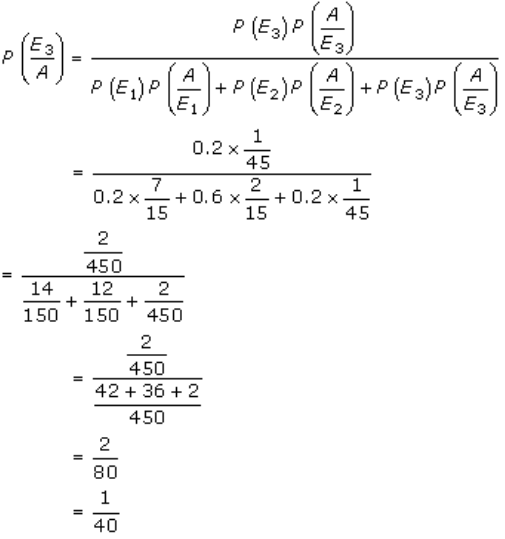Thus, the required probability is 1/40.

5. Solution:

Let’s consider the following events,

E1 – Getting 1 or 2 in a throw of die

E2 – Getting 3, 4, 5 or 6 in a throw of die

A – Getting exactly one tail

So clearly, we have

P(E1) = 2/6 = 1/3

P(E2) = 4/6 = 2/3

P(A/E1) = 3/8

P(A/E2) = ½

Now, the required probability is given by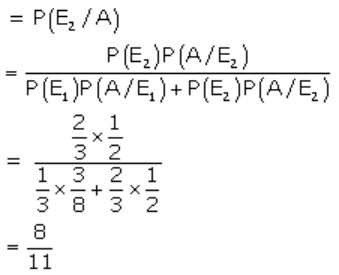6. Solution:

Let’s consider the following events,

E1 – First group wins

E2 – Second group wins

A – New product is introduced

It’s given that,

P(E1) = 0.6

P(E2) = 0.4

P(A/E1) = 0.7

P(A/E2) = 0.3

So, the required probability P(E2/A) is given by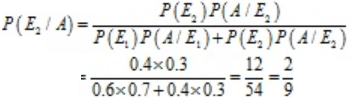Hence,

P(E2/A) = 2/9